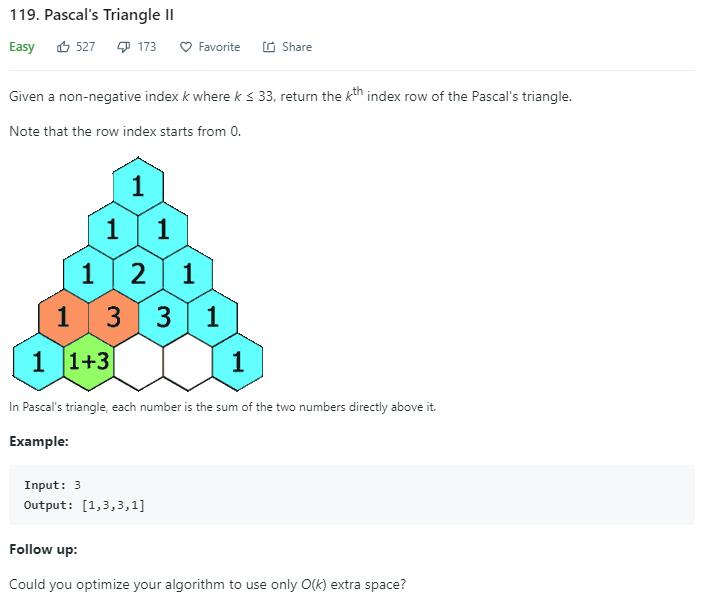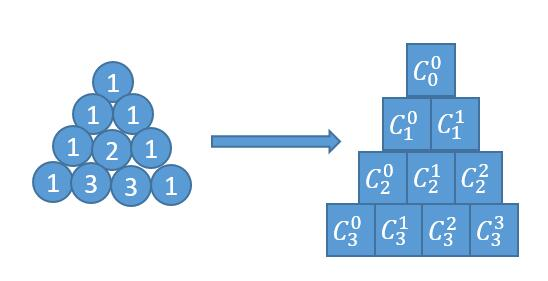# 题目描述（简单难度）118 题 一样，依旧是杨辉三角。区别在于之前是输出所有层的数，这道题只需要输出第 k 层的数。

# 解法一

118 题 一样，我们只需要一层一层的求。但是不需要把每一层的结果都保存起来，只需要保存上一层的结果，就可以求出当前层的结果了。

public List<Integer> getRow(int rowIndex) {
List<Integer> pre = new ArrayList<>();
List<Integer> cur = new ArrayList<>();
for (int i = 0; i <= rowIndex; i++) {
cur = new ArrayList<>();
for (int j = 0; j <= i; j++) {
if (j == 0 || j == i) {
} else {
}
}
pre = cur;
}
return cur;
}


public List<Integer> getRow(int rowIndex) {
int pre = 1;
List<Integer> cur = new ArrayList<>();
for (int i = 1; i <= rowIndex; i++) {
for (int j = 1; j < i; j++) {
int temp = cur.get(j);
cur.set(j, pre + cur.get(j));
pre = temp;
}
}
return cur;
}


public List<Integer> getRow(int rowIndex) {
int pre = 1;
List<Integer> cur = new ArrayList<>();
for (int i = 1; i <= rowIndex; i++) {
for (int j = i - 1; j > 0; j--) {
cur.set(j, cur.get(j - 1) + cur.get(j));
}
}
return cur;
}


# 解法二 公式法$C^k_n = n!/(k!(n-k)!) = (n*(n-1)*(n-2)*...(n-k+1))/k!$

public List<Integer> getRow(int rowIndex) {
List<Integer> ans = new ArrayList<>();
int N = rowIndex;
for (int k = 0; k <= N; k++) {
}
return ans;
}

private int Combination(int N, int k) {
long res = 1;
for (int i = 1; i <= k; i++)
res = res * (N - k + i) / i;
return (int) res;
}


$C_n^k=C_n^{k-1}\times(n-k+1)/k$

public List<Integer> getRow(int rowIndex) {
List<Integer> ans = new ArrayList<>();
int N = rowIndex;
long pre = 1;
for (int k = 1; k <= N; k++) {
long cur = pre * (N - k + 1) / k;